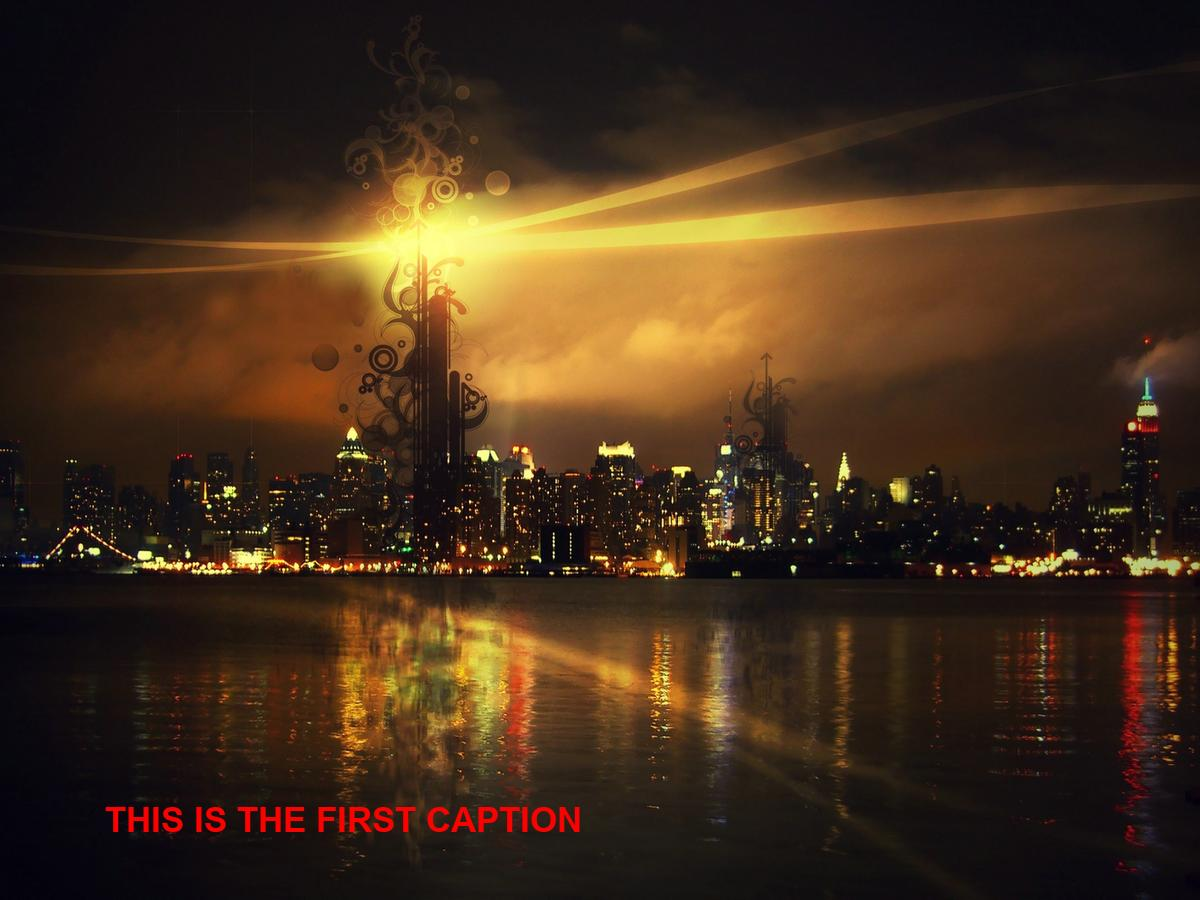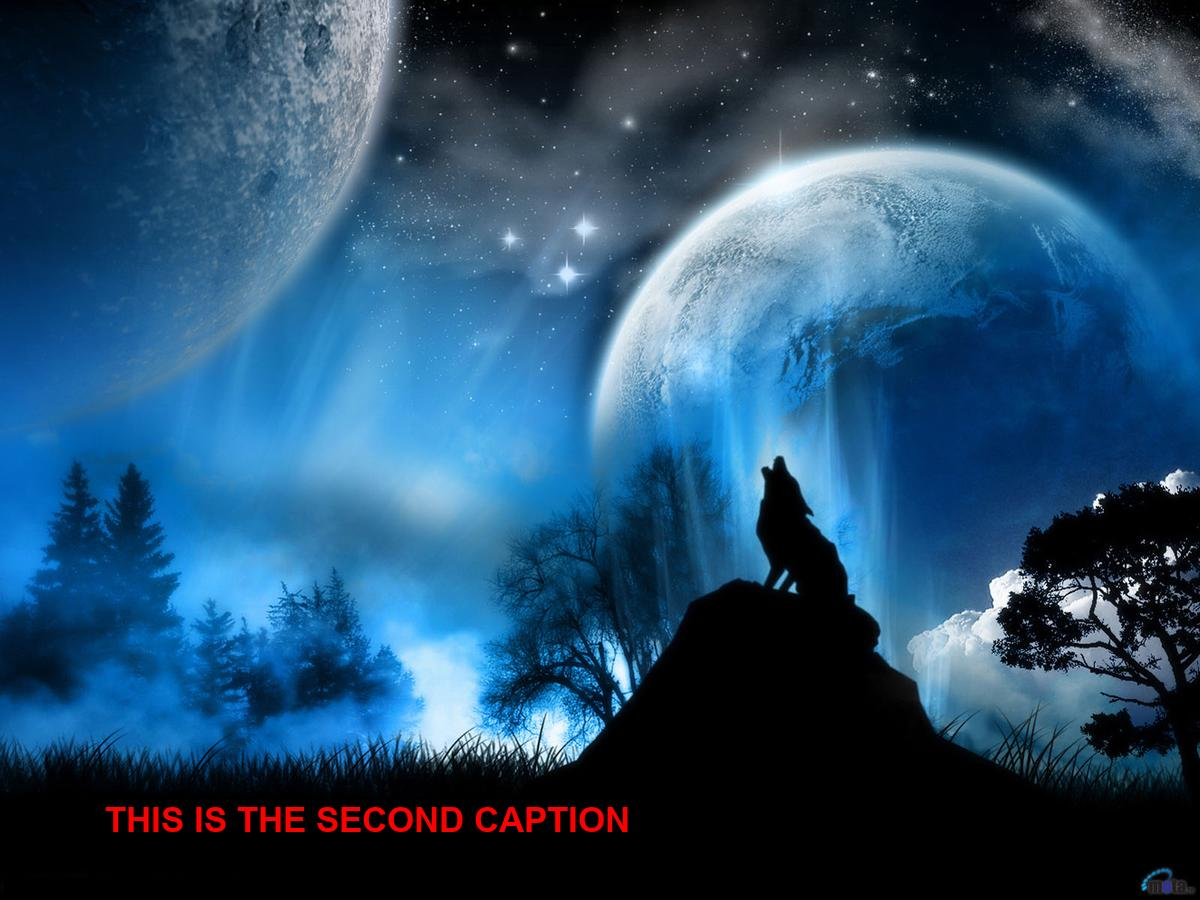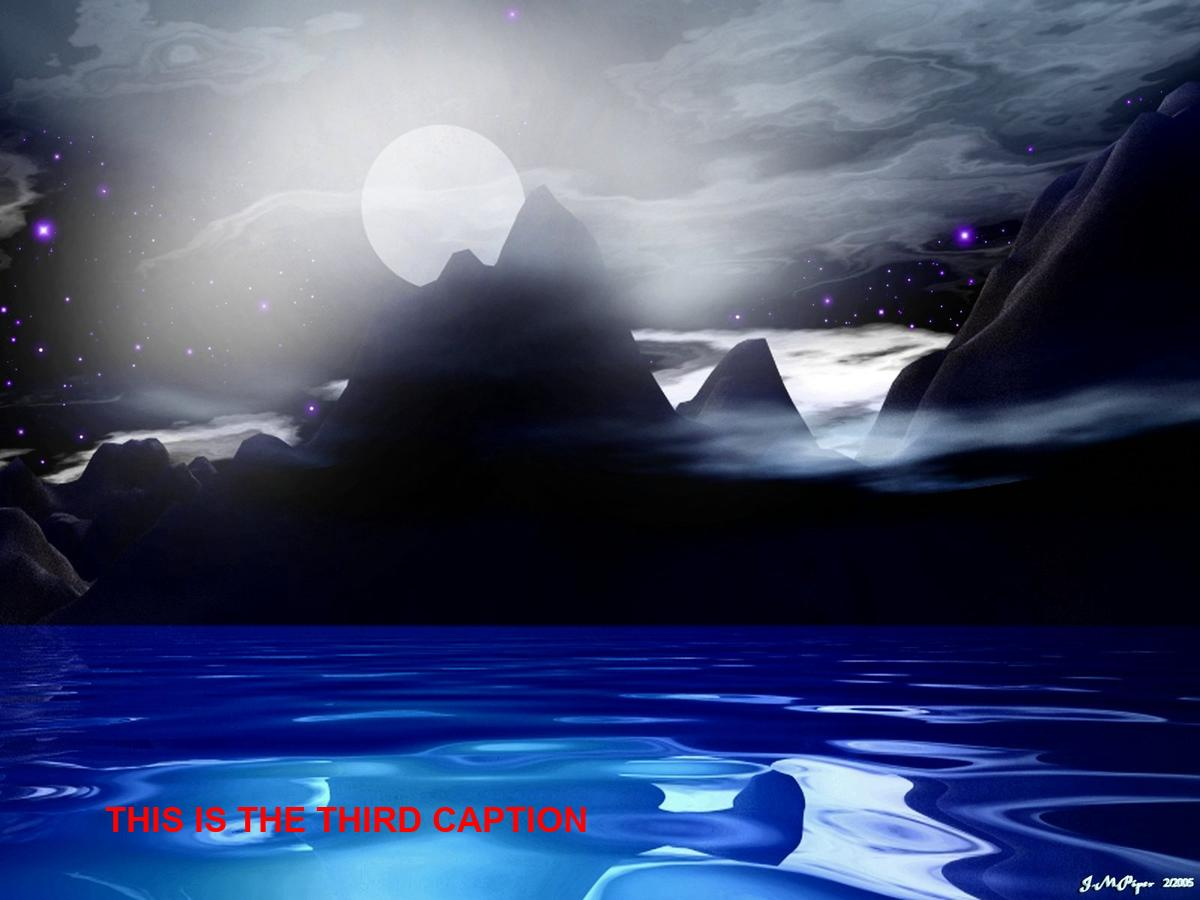# Image Captioning using Python

Image captioning is a very classical and challenging problem coming to Deep Learning domain, in which we generate the textual description of image using its property, but we will not use Deep learning here. In this article, we will simply learn how can we simply caption the images using PIL.

Preprocessing on images is a great utility provided by Python PIL library. Not only we can change size, mode, orientation but we can draw on images, write text over it as well.

Install the required libraries:

```urllib
requests
PIL
glob
shutil```

• Download the `font.ttf` file (before running the code) using this link.
• Make folder with name as “CaptionedImages” beforehand where the output captioned images will be stored.

Below is the stepwise implementation using Python:

Step #1:

 `# importing required libraries ` `import` `urllib ` `import` `requests ` `import` `os ` ` `  `# retrieving using image url  ` `urllib.request.urlretrieve(``"https://i.ibb.co/xY4DJJ5/img1.jpg"``, ``"img1.jpg"``) ` `urllib.request.urlretrieve(``"https://i.ibb.co/Gnd1Y1L/img2.jpg"``, ``"img2.jpg"``) ` `urllib.request.urlretrieve(``"https://i.ibb.co/Z6JgS1L/img3.jpg"``, ``"img3.jpg"``) ` ` `  `print``(``'Images downloaded'``) ` ` `  `# get current working directory path ` `path ``=` `os.getcwd() ` ` `  ` `  `captionarr ``=` `[ ` `    ``"This is the first caption"``, ` `    ``"This is the second caption"``, ` `    ``"This is the third caption"` `    ``] `

Step #2:

 `# importing necessary functions from PIL ` `from` `PIL ``import` `Image ` `from` `PIL ``import` `ImageFont ` `from` `PIL ``import` `ImageDraw  ` ` `  `# print(os.getcwd()) ` ` `  `# checking the file mime types if ` `# it is jpg, png or jpeg ` `def` `ext(``file``): ` `    ``index ``=` `file``.find(``".jpg"``) ` `    ``current_file ``=` `"" ` `    ``current_file ``=` `file``[index:] ` `    ``return` `current_file  ` ` `  `def` `ext2(``file``): ` `    ``index ``=` `file``.find(``".jpeg"``) ` `    ``current_file ``=` `"" ` `    ``current_file ``=` `file``[index:] ` `    ``return` `current_file  ` ` `  `def` `ext3(``file``): ` `    ``index ``=` `file``.find(``".png"``) ` `    ``current_file ``=` `"" ` `    ``current_file ``=` `file``[index:] ` `    ``return` `current_file  ` ` `  ` `  `# converting text from lowercase to uppercase ` `def` `convert(words): ` `    ``s ``=` `"" ` `    ``for` `word ``in` `words: ` `        ``s ``+``=` `word.upper()  ` `    ``return` `s ` ` `  `caption_first ``=` `convert(captionarr[``0``]) ` `caption_second ``=` `convert(captionarr[``1``]) ` `caption_third ``=` `convert(captionarr[``2``]) ` `     `  `print``(caption_first) ` `print``(caption_second) ` `print``(caption_third) ` ` `  ` `  `count ``=` `0` ` `  `for` `f ``in` `os.listdir(``'.'``): ` `    ``try``: ` `        ``# Checking for file types if jpg, png ` `        ``# or jpeg excluding other files ` `        ``if` `(ext(f) ``=``=` `'.jpg'` `or` `ext2(f) ``=``=` `'.jpeg'` `or` `ext3(f) ``=``=` `'.png'``): ` `            ``img ``=` `Image.``open``(f)  ` `            ``width, height ``=` `img.size ` `            ``basewidth ``=` `1200` `            ``# print(height) ` ` `  `            ``# Resizinng images to same width height ` `            ``wpercent ``=` `(basewidth ``/` `float``(img.size[``0``])) ` `            ``hsize ``=` `int``((``float``(img.size[``1``])``*``float``(wpercent))) ` `            ``img ``=` `img.resize((basewidth, hsize), Image.ANTIALIAS) ` `            ``new_width, new_height ``=` `img.size ` ` `  ` `  `            ``# print(new_height) ` `            ``# changing image mode if not in RGB ` `            ``if` `not` `img.mode ``=``=` `'RGB'``: ` `                ``img ``=` `img.convert(``'RGB'``) ` `         `  `            ``draw ``=` `ImageDraw.Draw(img) ` `            ``# font = ImageFont.truetype(, ) ` `            ``# initializing which font will be chosen by us ` `            ``font ``=` `ImageFont.truetype(``"Arial Bold.ttf"``, ``35``)  ` `             `  `             ``# First Caption on First image ` `            ``if` `count ``=``=` `0``: ` `                ``draw.text((new_width ``/` `15` `+` `25``, new_height ``-` `100``), ` `                           ``caption_first, (``255``, ``0``, ``0``), font ``=` `font, ` `                           ``align ``=``"center"``) ` `                            `  `            ``# Second Caption on Second image ` `            ``elif` `count ``=``=` `1``:  ` `                ``draw.text((new_width ``/` `15` `+` `25``, new_height ``-` `100``), ` `                          ``caption_second, (``255``, ``0``, ``0``), font ``=` `font, ` `                          ``align ``=``"center"``) ` `                                                   `  `            ``# Third Caption on Third image ` `            ``else``:  ` `                ``draw.text(( new_width ``/` `15` `+` `25``, new_height ``-` `100``), ` `                            ``caption_third, (``255``, ``0``, ``0``), font ``=` `font, ` `                            ``align ``=``"center"``)              ` ` `  `            ``img.save(``"CaptionedImges/{}"``.``format``(f))      ` `            ``print``(``'done'``) ` `            ``count ``=` `count ``+` `1` `             `  `    ``except` `OSError: ` `        ``pass`

Step #3:
Sorting the output files in accordance to last modified time so that they do not get placed in alphabetical or any other mismanaged order.

 `import` `os ` `import` `glob ` `import` `shutil ` ` `  `# changing directory to CaptionedImages ` `os.chdir(``".\\CaptionedImges"``)  ` ` `  `fnames ``=` `[] ` `for` `file` `in` `os.listdir(``'.'``): ` `    ``# appending files in directory to the frames arr ` `    ``fnames.append(``file``)  ` ` `  `# sorting the files in frames array  ` `# on the basis of last modified time ` `# reverse = True means ascending order sorting ` `fnames.sort(key ``=` `lambda` `x: os.stat(x).st_ctime, reverse ``=` `True``) `

Output:My Personal Notes arrow_drop_upCompetitive Programmer, Full Stack Developer, Technical Content Writer, Machine Learner

If you like GeeksforGeeks and would like to contribute, you can also write an article using contribute.geeksforgeeks.org or mail your article to contribute@geeksforgeeks.org. See your article appearing on the GeeksforGeeks main page and help other Geeks.

Please Improve this article if you find anything incorrect by clicking on the "Improve Article" button below.

Article Tags :

Be the First to upvote.

Please write to us at contribute@geeksforgeeks.org to report any issue with the above content.